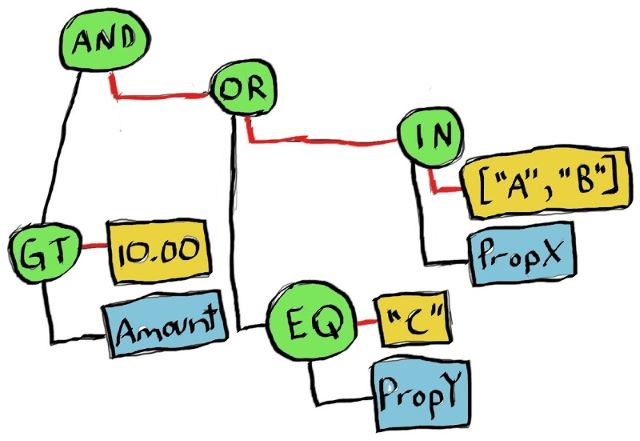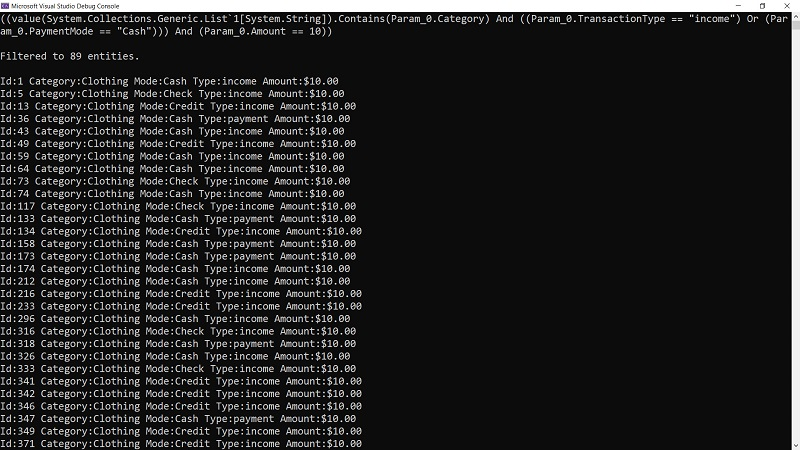## 动态构建LINQ表达式_linq 表达式 动态执行_寒冰屋的博客-程序员秘密

Transaction介绍

Lambda表达式和编译

LINQ意为语言集成查询。它提供了一种一致的强类型机制，用于跨各种源查询数据。LINQ基于表达式。本文通过在参考应用程序中构建自定义表达式树来探索LINQ和基础表达式。LINQ意为语言集成查询，是我最喜欢的.NETC＃技术之一。使用LINQ，开发人员可以直接在强类型代码中编写查询。LINQ提供了跨数据源一致的标准语言和语法。

# 基础

``````using System;
using System.Linq;
public class Program
{
public static void Main()
{
var someNumbers = new int[]{4, 8, 15, 16, 23, 42};
var query = from num in someNumbers
where num > 10
orderby num descending
select num.ToString();
Console.WriteLine(string.Join('-', query.ToArray()));
// 42-23-16-15
}
}``````

``````var secondQuery = someNumbers.Where(n => n > 10)
.OrderByDescending(n => n)
.Select(n => n.ToString());``````

1. 设置了一个数据源，称为提供程序，以使查询根据该数据源进行操作。例如，到目前为止显示的代码使用内置的LINQ to Objects提供程序。EF Core项目使用映射到数据库的EF Core提供程序
2. 定义查询并将其转换为表达式树。一会儿我将介绍更多表达式。
3. 执行查询，并返回数据。

``````var found = false;
foreach(var item in secondQuery.AsEnumerable())
{
if (item == "23")
{
found = true;
break;
}
}``````

# 挑战

``````{
"condition":"and",
"rules":[
{
"label":"Category",
"field":"Category",
"operator":"in",
"type":"string",
"value":[
"Clothing"
]
},
{
"condition":"or",
"rules":[
{
"label":"TransactionType",
"field":"TransactionType",
"operator":"equal",
"type":"boolean",
"value":"income"
},
{
"label":"PaymentMode",
"field":"PaymentMode",
"operator":"equal",
"type":"string",
"value":"Cash"
}
]
},
{
"label":"Amount",
"field":"Amount",
"operator":"equal",
"type":"number",
"value":10
}
]
}``````

# 解决方案：动态表达式

JeremyLikness/ExpressionGenerator

``````var jsonStr = File.ReadAllText("rules.json");
var jsonDocument = JsonDocument.Parse(jsonStr);``````

``````                        /-----------AND-----------\
|                         |
/-AND-\                      |
Category IN ['Clothing']   Amount eq 10.0        /-OR-\
TransactionType EQ 'income'  PaymentMode EQ 'Cash'``````

# Transaction介绍

``````public class Transaction
{
public int Id { get; set; }
public string Category { get; set; }
public string TransactionType { get; set; }
public string PaymentMode { get; set; }
public decimal Amount { get; set; }
}``````

# 参数表达式

main方法返回一个谓词函数。这是开始的代码：

``````public Func<T, bool> ParsePredicateOf<T>(JsonDocument doc)
{
var itemExpression = Expression.Parameter(typeof(T));
var conditions = ParseTree<T>(doc.RootElement, itemExpression);
}``````

``var query = ListOfThings.Where(t => t.Id > 2);``

t =>是所述第一参数和表示一个项目的列表中的类型。因此，我们为该类型创建一个参数。然后，我们递归地遍历JSON节点以构建树。

# 逻辑表达式

``````private Expression ParseTree<T>(
JsonElement condition,
ParameterExpression parm)
{
Expression left = null;
var gate = condition.GetProperty(nameof(condition)).GetString();

JsonElement rules = condition.GetProperty(nameof(rules));

Binder binder = gate == And ? (Binder)Expression.And : Expression.Or;

Expression bind(Expression left, Expression right) =>
left == null ? right : binder(left, right);``````

``private delegate Expression Binder(Expression left, Expression right);``

binder变量仅设置顶级表达式：Expression.AndExpression.Or。两者都采用左右表达式来求值。

bind函数更加有趣。遍历树时，我们需要构建各个节点。如果尚未创建表达式（leftnull），则从创建的第一个表达式开始。如果我们有一个现有的表达式，则可以使用该表达式合并这两个方面。

``foreach (var rule in rules.EnumerateArray())``

# 属性表达式

``````string @operator = rule.GetProperty(nameof(@operator)).GetString();
string type = rule.GetProperty(nameof(type)).GetString();
string field = rule.GetProperty(nameof(field)).GetString();
JsonElement value = rule.GetProperty(nameof(value));
var property = Expression.Property(parm, field);``````

``````var filter = new List<string> { "Clothing" };
Transactions.Where(t => filter.Contains(t.Category));``````

# 常量和调用表达式

``````private readonly MethodInfo MethodContains = typeof(Enumerable).GetMethods(
BindingFlags.Static | BindingFlags.Public)
.Single(m => m.Name == nameof(Enumerable.Contains)
&& m.GetParameters().Length == 2);``````

``````if (@operator == In)
{
var contains = MethodContains.MakeGenericMethod(typeof(string));
object val = value.EnumerateArray().Select(e => e.GetString())
.ToList();
var right = Expression.Call(
contains,
Expression.Constant(val),
property);
left = bind(left, right);
}``````

• 调用方法（contains
• 作为要检查的参数的值（带有ClothingExpression.Constant(val)的列表）
• 要针对（t.Category）进行检查的属性。

``Transactions.Where(t => (new List<string> { "Clothing" }).Contains(t.Category));``

``````if (rule.TryGetProperty(nameof(condition), out JsonElement check))
{
var right = ParseTree<T>(rule, parm);
left = bind(left, right);
continue;
}``````

``Transactions.Where(t => (new List<string> { "Clothing" }).Contains(t.Category) && <something>);``

# 比较表达式

``````object val = (type == StringStr || type == BooleanStr) ?
(object)value.GetString() : value.GetDecimal();
var toCompare = Expression.Constant(val);
var right = Expression.Equal(property, toCompare);
left = bind(left, right);``````

``Transactions.Where(t => t.TransactionType == "income");``

``Transactions.Where(t => t.TransactionType == "income" || t.PaymentMode == "Cash");``

``````(
(value(System.Collections.Generic.List`1[System.String]).Contains(Param_0.Category)
And (
(Param_0.TransactionType == "income")
Or
(Param_0.PaymentMode == "Cash"))
)
And
(Param_0.Amount == 10)
)``````

# Lambda表达式和编译

``````var conditions = ParseTree<T>(doc.RootElement, itemExpression);
if (conditions.CanReduce)
{
conditions = conditions.ReduceAndCheck();
}
var query = Expression.Lambda<Func<T, bool>>(conditions, itemExpression);
return query.Compile();``````

``````var predicate = jsonExpressionParser
.ParsePredicateOf<Transaction>(jsonDocument);
var transactionList = Transaction.GetList(1000);
var filteredTransactions = transactionList.Where(predicate).ToList();
filteredTransactions.ForEach(Console.WriteLine);``````# 从内存到数据库

``````public Func<T, bool> ParsePredicateOf<T>(JsonDocument doc)
{
var query = ParseExpressionOf<T>(doc);
return query.Compile();
}``````

ExpressionGenerator程序使用编译后的查询。DatabaseTest使用原始λ表达式。它将其应用于本地SQLite数据库，以演示EF Core如何解析该表达式。在将1000transactions创建并插入数据库后，代码将检索count

``````var count = await context.DbTransactions.CountAsync();
Console.WriteLine(\$"Verified insert count: {count}.");``````

``````SELECT COUNT(*)
FROM "DbTransactions" AS "d"``````

``````var parser = new JsonExpressionParser();
var predicate = parser.ParseExpressionOf<Transaction>(
JsonDocument.Parse(
var query = context.DbTransactions.Where(predicate)
.OrderBy(t => t.Id);
var results = await query.ToListAsync();``````

``````SELECT "d"."Id", "d"."Amount", "d"."Category", "d"."PaymentMode", "d"."TransactionType"
FROM "DbTransactions" AS "d"
WHERE ("d"."Category" IN ('Clothing') &
((("d"."TransactionType" = 'income') AND "d"."TransactionType" IS NOT NULL) |
(("d"."PaymentMode" = 'Credit') AND "d"."PaymentMode" IS NOT NULL))) &
("d"."Amount" = '10.0')
ORDER BY "d"."Id"``````

# 结论

LINQ表达式是过滤和转换数据的非常强大的工具。我希望该示例有助于揭开表达式树的构建方式。当然，解析表达式树感觉有点像魔术。Entity Framework Core如何遍历表达式树以产生有意义的SQL？我正在自己探索这个问题，并在我的朋友ExpressionVisitor帮助下进行了探索。

### 计算机专业应该掌握的数学知识_计算机类专业应该掌握那些数学_王小胖a的博客-程序员秘密

http://blog.sina.com.cn/s/blog_6233611f0100m4vs.html

### 跨语言检索的QA（google research EMNLP 2020）_lareqa github_我黑切呢**的博客-程序员秘密

LAReQA: Language-Agnostic Answer Retrieval from a Multilingual Pool trained models are available at https://tfhub.dev/s?q=lareqa. dataset and evaluation code are available at https://github.com/google-research-datasets/lareqa.提出一个Language-Agnostic的检..

### FZU 2135 数字游戏（思维题）_夜幕下的ACM之路的博客-程序员秘密

Problem Description给一个N位的正整数，该数不包含前导0，先让你调整其中每个数字的位置，得到另一个n位的数，并且使得这个数越小越好，而且这个数不能包含前导0。比如543210可以变成102345，而12345保持不变才是最优结果。Input第一行一个整数T（T<=100)，表示有T组数据。每组数据先输入一行一个整数N(1<=N<=100)，表示位数，接下来一行输入一个N位的不包含前

### C语言利用联合体和结构体位域实现位带操作_结构体位域操作 联合体_cai_niao321的博客-程序员秘密

C语言利用联合体和结构体位域实现位带操作代码C语言编程时经常需要操作特定的数据中特定的bit位，可以通过与、或操作实现。我试了一下用结构体位域和联合体，实现了可单独对bit操作。和正常变量赋值一样，即可对单独的bit位写1或写0。下面是代码和运行结果。小白的第一篇博客，工作时遇到的问题用博客记录一下，方便以后查看。希望能坚持下去。代码#include "stdio.h"typedef...

flink sql 读取kafka 关联mysql维表 , 写出到pg中 简单演示

### \$project_zangodb_C-haidragon的博客-程序员秘密

\$project修改输入文档的结构，如重命名、增加、删除字段、创建计算结果例1：查询学生的姓名、年龄db.stu.aggregate([ {\$project:{_id:0,name:1,age:1}}])例2：查询男生、女生人数，输出人数db.stu.aggregate([ {\$group:{_id:'\$gender',counter:{\$sum:1}}

### Vue 中使用Mock.js生成模拟数据_没心没肺可苦可乐的博客-程序员秘密

Mock JS最近在做一个平台报表分析系统的前端界面，就是用一堆图表来展示什么点击量啊、在线人数啊、用户构成等等数据，然而后端还没开始实现调用这些数据的功能接口，于是只能用假数据了。一开始是用的本地json文件来模拟数据，虽然图表效果出来了，但是不够灵活，看着就很假，于是心血来潮，想试着用一下以前不知道在哪听来的mock.j...